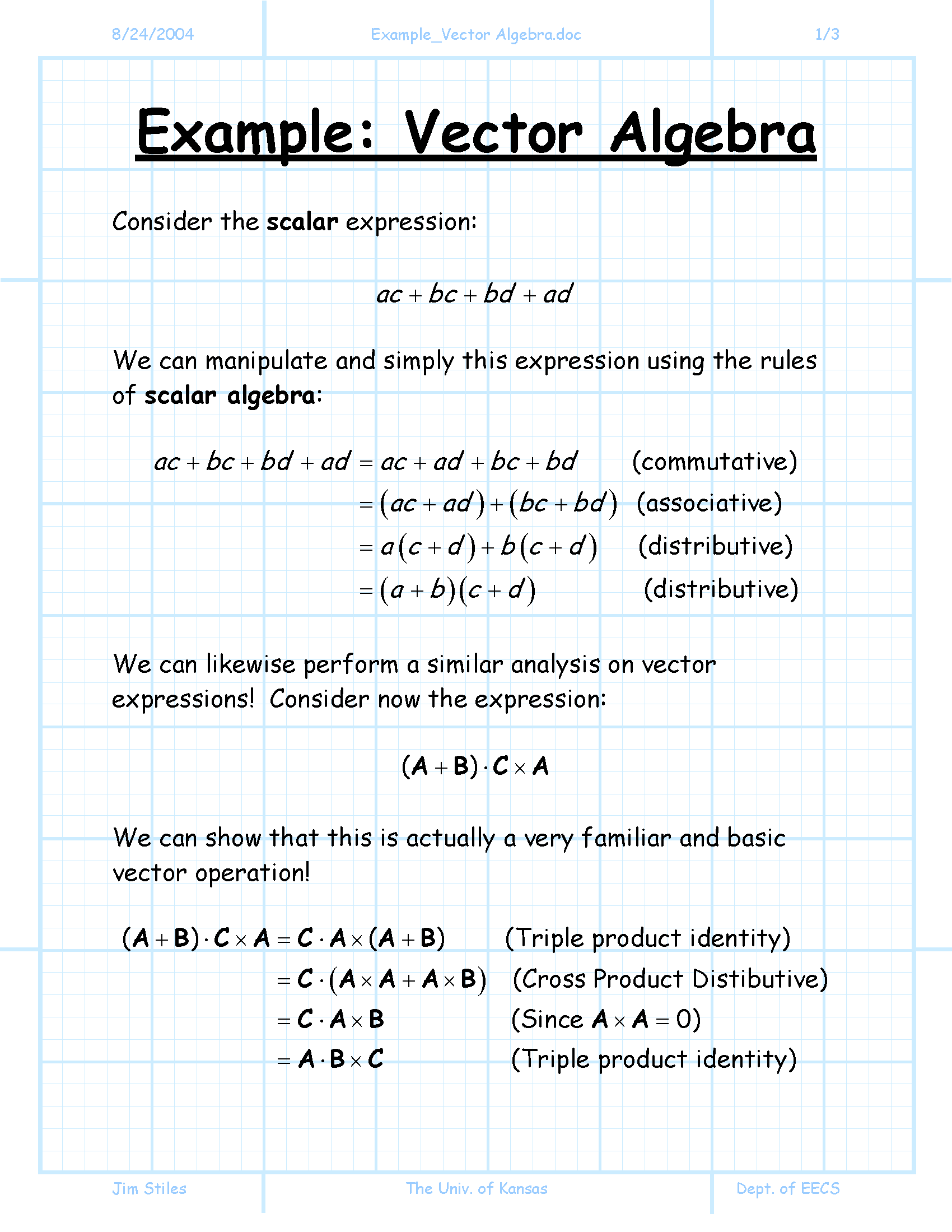Yelizaveta ZavesovaNumber Line and Negative Number

Objective:    Students will be able to locate negative numbers on a number line, and compare them.

Number line is a horizontal line composed of an origin and tick marks (units) of equal distance. The origin divides the number line into two regions: the region to the right of the origin is the positive side; the region to the left of the origin is the negative side. On a number line, every number is paired with a point. The number is known as the coordinate of the point; the point is known as the graph of the number.For instance, the number -5 is graphed and labeled as the point P. Point P is located 5 units from the left of the origin O. -5 is the coordinate of P; P is the graph of -5.In addition, the smaller a negative number, the farther it is from the origin. Hence, -7 is less than -6.8 because -7 is farther from the origin than -6.8.Example 1: Find the coordinates of points A, B, and C, and compare the numbers.Answer for Example 1:

Coordinate of Point A is -1/2 or -0.5
Coordinate of Point B is -3
Coordinate of Point C is -4 3/4 or -4.75

- 1/2 > -3 > -4 3/4

Example 2: Graph the coordinates -1.5, -2 2/3, and -7.4, and labeled them as X, Y, and Z accordingly.

Answer to Example 2:Contact:Copyright © 2022 Bancroft Middle School